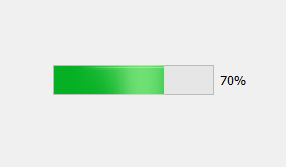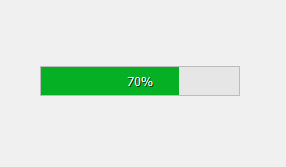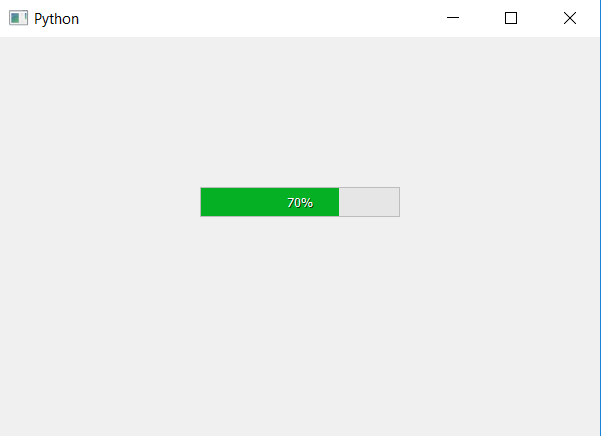Open in App
Not now

# PyQt5 | How to set percentage indicator in middle of Progress Bar ?

• Last Updated : 22 Apr, 2020

In this article we will see how to set the percentage indicator inside the progress bar. When we create a progress bar the percentage indicator is by default is at right hand side of the progress bar i.e outside the progress bar.

Below is how normal percentage indicator looks like vs how it look inside it.In order to do this we will do the following :

1. Create a progress bar.
2. Set its alignment to center

In order to change the alignment of progress bar we will use `setAlignment` method.

 `# importing libraries``from` `PyQt5.QtWidgets ``import` `*` `from` `PyQt5 ``import` `QtCore``from` `PyQt5.QtGui ``import` `*` `from` `PyQt5.QtCore ``import` `*` `import` `sys`` ` ` ` `class` `Window(QMainWindow):``    ``def` `__init__(``self``):``        ``super``().__init__()`` ` `        ``# setting title``        ``self``.setWindowTitle(``"Python "``)`` ` `        ``# setting geometry``        ``self``.setGeometry(``100``, ``100``, ``600``, ``400``)`` ` `        ``# calling method``        ``self``.UiComponents()`` ` `        ``# showing all the widgets``        ``self``.show()`` ` `    ``# method for widgets``    ``def` `UiComponents(``self``):`` ` `        ``# creating progress bar``        ``bar ``=` `QProgressBar(``self``)`` ` `        ``# setting geometry to progress bar``        ``bar.setGeometry(``200``, ``150``, ``200``, ``30``)`` ` `        ``# set value to progress bar``        ``bar.setValue(``70``)``         ` `        ``# changing the alignment of progress bar``        ``bar.setAlignment(QtCore.Qt.AlignCenter)`` ` ` ` `# create pyqt5 app``App ``=` `QApplication(sys.argv)`` ` `# create the instance of our Window``window ``=` `Window()`` ` `# start the app``sys.exit(App.``exec``())`

Output :My Personal Notes arrow_drop_up